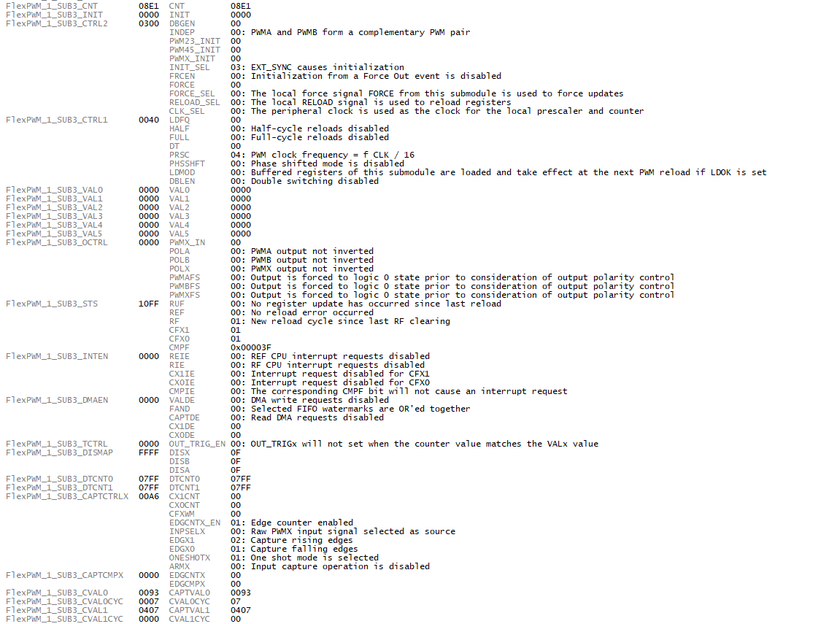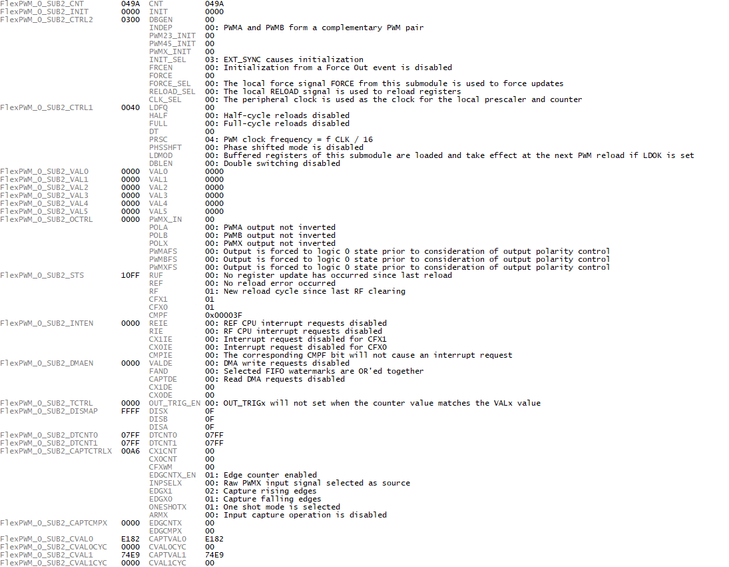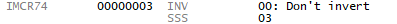# MPC5744P FLEXPWM1_X3 capture counter seems to overflow before 0xFFFF

cancel
Showing results for
Show  only  | Search instead for
Did you mean:

## MPC5744P FLEXPWM1_X3 capture counter seems to overflow before 0xFFFF

350 ViewsContributor III

Hello

MPC5744P 257BGA (1N15P), I have 4 identical circuits in my application that are mapped to PWMX inputs in order to measure pulse width from hall sensors. All of them are configured the same way.

Prescaler is configured /16, and with FPERIPH 160Mhz the counter yields 6553,6us full scale.

``````typedef struct {
volatile FlexPWM_SUB_tag* pwmreg;
uint8_t is_mscr_configurable;
uint8_t pin_mscr;
uint8_t pin_imcr;
uint8_t pin_sss;
uint8_t pwm_module;
uint8_t pwm_submodule;
}tPULS_SENSH_DATA;

const tPULS_SENSH_DATA puls_hall_fast_cfg[N_SENS_HALL] = {
{&(FlexPWM_0.SUB), 0, 60, 93, 2, 0, 1}, //H1A
{&(FlexPWM_0.SUB), 0, 101, 99, 3, 0, 3}, //H1B
{&(FlexPWM_0.SUB), 0, 98, 96, 2, 0, 2}, //H2A
{&(FlexPWM_1.SUB), 1, 125, 74, 1, 1, 3} //H2B
};

for(i = 0; i < N_SENS_HALL; i++) {
p_pwm = puls_hall_fast_cfg[i].pwmreg;

if(puls_hall_fast_cfg[i].is_mscr_configurable) {
SIUL2.MSCR[puls_hall_fast_cfg[i].pin_mscr].B.SSS = puls_hall_fast_cfg[i].pin_sss;
} else {
SIUL2.IMCR[puls_hall_fast_cfg[i].pin_imcr].B.SSS = puls_hall_fast_cfg[i].pin_sss;
}
SIUL2.MSCR[puls_hall_fast_cfg[i].pin_mscr].B.IBE = 1;
SIUL2.MSCR[puls_hall_fast_cfg[i].pin_mscr].B.OBE = 0;

p_pwm->CTRL1.R = 0;
p_pwm->CTRL1.B.PRSC = 4;//4; // FCLK/16
p_pwm->CTRL2.R = 0;
p_pwm->CTRL2.B.INIT_SEL = 3;
p_pwm->INIT.R = 0;

p_pwm->CAPTCTRLX.B.CFXWM = 0;
p_pwm->CAPTCTRLX.B.EDGCNTX_EN = 1;
p_pwm->CAPTCTRLX.B.INPSELX = 0;
p_pwm->CAPTCTRLX.B.EDGX1 = 2; //Rising edges
p_pwm->CAPTCTRLX.B.EDGX0 = 1; //Falling edges
p_pwm->CAPTCTRLX.B.ONESHOTX = 1;

memset(&(puls_params_hall[i]), 0, sizeof(tPULS_PARAMS_HALL));
puls_params_hall[i].edge_counter = (uint8_t)(p_pwm->CAPTCMPX.B.EDGCNTX);

p_pwm->CAPTCTRLX.B.ARMX = 1; //Primera lectura

switch(puls_hall_fast_cfg[i].pwm_module) {
case 0:
default:
FlexPWM_0.MCTRL.B.LDOK |= ((1 << puls_hall_fast_cfg[i].pwm_submodule) & 0xf);
FlexPWM_0.MCTRL.B.RUN |= ((1 << puls_hall_fast_cfg[i].pwm_submodule) & 0xf);
break;
case 1:
FlexPWM_1.MCTRL.B.LDOK |= ((1 << puls_hall_fast_cfg[i].pwm_submodule) & 0xf);
FlexPWM_1.MCTRL.B.RUN |= ((1 << puls_hall_fast_cfg[i].pwm_submodule) & 0xf);
break;
}

}``````

``````for(i = 0; i < N_SENS_HALL; i++)
{
pwm_reg_ptr = puls_hall_fast_cfg[i].pwmreg;
hall_ptr = &(puls_params_hall[i]);
hall_ptr->istimeout_ctr++;
hall_edge_counter = (uint8_t)(pwm_reg_ptr->CAPTCMPX.B.EDGCNTX);
if(hall_edge_counter != hall_ptr->edge_counter) {
hall_ptr->istimeout_ctr = 0;
}
hall_ptr->edge_counter = hall_edge_counter;
if(hall_ptr->istimeout_ctr >= hall_ptr->timeoutctr_max) {
hall_ptr->istimeout_ctr = hall_ptr->timeoutctr_max;
hall_ptr->hall_ok = 0;
pwm_reg_ptr->CAPTCTRLX.B.ARMX = 1; //Retry
} else {
hall_ptr->hall_ok = 1;
}
if((pwm_reg_ptr->STS.R & 0xC0) == 0xC0) {
pwm_reg_ptr->STS.R |= 0xC0;
capt1_fast = pwm_reg_ptr->CVAL0.R;
capt2_fast = pwm_reg_ptr->CVAL1.R;
hall_ptr->istimeout_ctr = 0;
if(capt2_fast >= capt1_fast) {
capt_diff = capt2_fast - capt1_fast;
} else {
capt_diff = (0xFFFF - capt1_fast) + capt2_fast;
}
hall_ptr->pwidth_us = capt_diff * K_SENSH_PWIDTH_TO_US;
pwm_reg_ptr->CAPTCTRLX.B.ARMX = 1; //New reading armed
}
}``````

Now, the first 3 work fine, but the 4th (FLEXPWM1_X3) does not work.

The symptoms:

- CAPTVAL1 - CAPTVAL0 = 1501 LSB all the time, irrespective of the PRESCALER configuration.

- The counter does not increment beyond 0x1000

This is the register snapshot. The CAPTVAL1 - CAPTVAL0 should deliver near 37000 LSB. Notice CNT under 0x1000.Meanwhile, the same device connected to the input #2 (FLEXPWM0_X2) has this register snapshot. Notice that the CNT register is well beyond 0x1000. And capture difference yields 37000.Regarding the FLEXPWM1_X3 pin, MSCR125.SSS = 1:IMCR74 is configured in another code part (for another pin), but I understand it has no relevance here.FlexPWM1 configurationAnd FlexPWM0 to compareI am completely lost on this one. I don't understand how under the same configuration,  FLEXPWM0 {1, 2, 3} are able to count 0000...FFFF, but on the other hand, FLEXPWM1 {3} is not able to count beyond 0x1000 (I didn't test the actual maximum value it overflows, but checking it real time with TRACE32, I can guarantee that at least).

Tags (3)
0 Replies